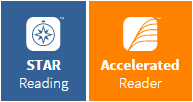AR reading quizzes should not be taken outside the district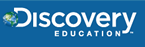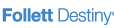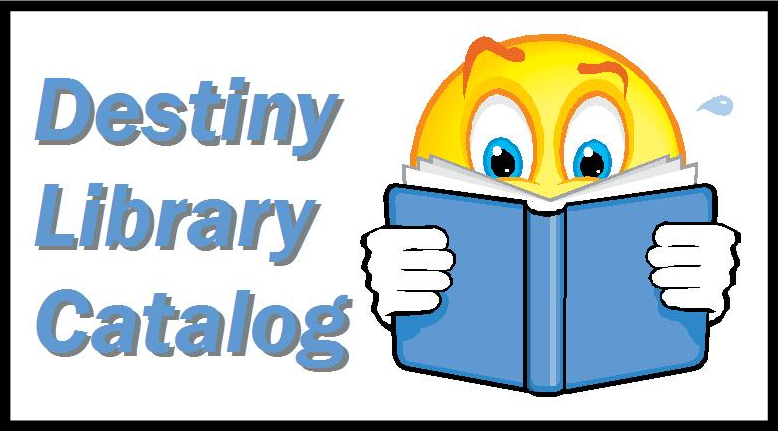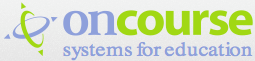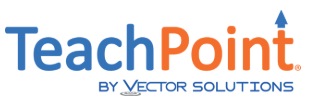GPS Windows 10-Tips and Strategies

Math Sites 4 Fun & Practice:

Annenberg Learner (K-12)-Mathematics

https://www.learner.org/subject/mathematics/

NCTM: Illuminations Lessons (http://illuminations.nctm.org/) All Grades, Pre-K-2, 3-5, 6-8, 9-12. All Standards, Number & Operations, Algebra, Geometry. Resources galore, including 63 Online activities, 487 lessons, and hundreds of Web links. For example in Turtle Pond, students guide a turtle into a pond using angles and measurements

AAA Math (http://www.aaamath.com/) Basic Math Skills for K-5. Topics include: Addition, Algebra, Comparing, Counting, Decimals, Division, Equations, Estimation, Exponents, Fractions, Geometry, Graphs, Measurement, Mental, Math, Money, Multiplication, Naming, Numbers, Patterns, Percent, Place Value, Practical Math, Properties, Ratios, Statistics, and Subtraction.

Microsoft Calculator Plus (https://www.microsoft.com/en-us/store/apps/calculator-plus/9wzdncrdhjwd) A complete replacement for the Windows calculator plus so much more. Use it for addition, subtraction, multiplication and division. It can also fill in for a scientific calculator for trigonometric functions, logarithms, logic, and statistics. Plus, it includes a unit converter. Requires a PC running Microsoft Windows.

10 Good Video Sources for Math Students and Teachers

http://www.freetech4teachers.com/2013/04/10-good-video-sources-for-math-students.html#.Vdy1k5c5CQw

High school math, fun number facts, teachers teaching math to students and more.

Misunderstood Minds: Basics of Mathematics

(http://www.pbs.org/wgbh/misunderstoodminds/mathbasics.html) Interesting perspective on brain functions involved when children play with numbers. Includes several hands-on examples. Discusses Math and Memory, Math Attention, Temporal-Sequential Ordering, and the Developing Math Student. Be sure to click the links to Difficulties with Mathematics and Responses to the Problem for strategies to try with students.

Printable Math Activities and Math Help

(http://www.999inks.co.uk/Printable-Math-Activities-and-Math-Help.html). Printable math resources, geometry, algebra, calculus, number theory for elementary grades, plus special authentic math activities.

Microsoft Mathematics 4.0 for Windows

(
https://www.microsoft.com/en-us/download/details.aspx?id=15702) Free graphing calculator that plots in 2D and 3D. Offers step-by-step equation solving and other tools to help students with math and science studies.

GeoGebra Tutorials (https://wiki.geogebra.org/en/Tutorials)

Math Snacks (http://mathsnacks.org/). Short animations and mini-games designed to present mathematics in a very different way. Project of New Mexico State University, currently funded by a K-12 Discovery Learning grant from the National Science Foundation

Create Your Own Math Quiz http://www.quizzes.cc/

Great Graphing Resources Grades 1-8 http://www.startlocal.com.au/articles/educational_graphs.html

Elementary

MathLine Lessons Plans with Video for Grades PreK-2 (http://www.pbs.org/teachersource/mathline/lessonplans/search_k-2.shtm) Video based math tutorials for teachers. You pick the topic, watch the clip, match to MA state standards, read the lesson plan and more. Great selection of topics. Web sites available for grades 3-5 too.

ArithmAttack (http://www.sanchezclass.com/arithmattack.htm) A site for quick math games, facts, flashcards, multiplication tables. How many answers can you get correct in one minute. (Standard 3.n.9. Know multiplication facts through 10 x 10 and related division facts, e.g., 9x8=72 and 72)

Count us In (http://www.abc.net.au/countusin/default.htm) A site for elementary students that teaches number recognition, counting, patterns, etc. Consists of interactive games, etc. These games help children understand basic number concepts.

Kid's Place: Houghton Mifflin-Mathematics (http://www.eduplace.com/kids/mhm/index.html) Houghton Mifflin mathematics site, teaching math concepts through fun activities and interactives. Students take tests, do test prep and crunch numbers through brain teasers.

NCTM Illuminations (https://illuminations.nctm.org/) Hosted by the National Coalition of Teaching Mathematics Organization and dedicated to improving math education for students in grades PreK-12, this site boasts math games, activities, lessons, standards, and Web links to other great math sites. Especially wonderful for learning shapes and geometry. Probability and ratio is also impressive for even young students.

Project Interactivate for Elementary Grades 3-5 (http://www.shodor.org/interactivate/elementary/index.html) Java-based interactives to helps elementary school students explore science and mathematics. The activities are aligned to popular textbooks and to NCTM and NCEE standards. Concepts targeted include: Number and Operation, Geometry and Measurement, Function and Algebra, and Probability and Data Analysis.

My Math Sites (http://sites.google.com/site/mymathsites/home) Primary through Grade 7 listed by topic. Choose an area, then visit the collection of thematic links. Some lessons, some games.

Mobile Math (http://www.barcodesinc.com/articles/mobile-math-list.htm). Several Web sites with interactive games to teach a variety of math skills

Middle School

Figure This Math Challenges for Families (http://www.figurethis.org/video/video.htm#) Explore the site but don't forget to check out the Math Index page (http://www.figurethis.org/challenges/math_index.htm) containing math links by categories such as:

• Algebra: Equations, linear, Functions, Compare & Contrast, Graphs of Lines , Modeling Problems, Patterns, Problem Solving using Symbols, Rate of Change, Representations, Variable,

• Geometry: 2-D and 3-D figures, properties, , 2-D representations of 3-D figures, Connections to other disciplines, Coordinate Geometry, Geometric models of algebraic concepts, Induction and deduction, Networks, Scale Drawing, Similarity/Congruence, Transformations,

• Measurement: Angles and Length, Area and Circumference, Estimation, Metric and Customary Units , Rates and Velocity, Scale factors , Surface Area & Volume, Units,

• Number: Compare and order decimals, fractions, percents, Factors, Integers , Large numbers and notation , Percentages >100 and <1, Ratio and proportions, Solve problems with decimals, fractions, percents; Statistics & Probability Averages, Centers and Spreads , Collecting Data , Complementary and mutually exclusive events , Formulate Questions, Graphical representations of data, Make conjectures about differences , Make conjectures about relationships , Probability.

Activities (http://www.shodor.org/interactivate/activities/index.html) Interactive math problem-solving (Java applets) for several topics in Middle School Math, including Number and Operation Concepts (http://www.shodor.org/interactivate/activities/index.html#num), Geometry and Measurement Concepts (http://www.shodor.org/interactivate/activities/index.html#geo), Function and Algebra Concepts (http://www.shodor.org/interactivate/activities/index.html#fun), and Probability and Data Analysis Concepts (http://www.shodor.org/interactivate/activities/index.html#pro)

MathLine Lessons Plans with Video for Middles School (grades 6-8) (http://www.pbs.org/teachersource/mathline/lessonplans/search_k-2.shtm ) Video based math tutorials for teachers. You pick the topic, watch the clip, match to MA state standards, read the lesson plan, and more. Great selection of topics.

Math 6 Spy Guys, Alberta Dept. of Education (http://www.learnalberta.ca/content/mesg/html/math6web/index.html?page=home)

High School

Math in the News (http://www.ams.org/mathmedia/) American Mathematical Society collects news stories about math in the real world, including image of the month. Some stories are really obscure, but some are totally awesome!

Welcome to Calculus on the Web (http://www.math.temple.edu/~cow/) Linear algebra, number theory, abstract algebra, pre-calculus and calculus problems

Algebra Exercises, Problems & Quiz Activities (http://www.syvum.com/squizzes/algebra/) Practice makes perfect. Learning materials and puzzles galore!

Cornell Theory Center Math/Science Gateway (https://www.cac.cornell.edu/education/SAGE.aspx) Links to resources in mathematics and science for educators and students in grades 9-12.

Web2.0calc (http://web2.0calc.com/)

Free Web-based Scientific Calculator that can do most of what a high school student needs to do.

The Math Forum - Math Library - High School (http://mathforum.org/library/levels/high/) Math activities for a variety of topics, including algebra, pre-calculus, probability, early math, and problem of the week…so how many different ways can Wendy use 31 legs to build wooden dollhouse furniture?

MathLine Lessons Plans with Video for High School (grades 9-12) (http://www.pbs.org/teachersource/mathline/lessonplans/search_k-2.shtm ) Video based math tutorials for teachers. You pick the topic, watch the clip, match to MA state standards, read the lesson plan and more. Great selection of topics!

TeachersFirst Resource Listings-Math (http://www.teachersfirst.com/tchr-subj-date.cfm?subject=math&lower=9&upper=12) A variety of math teaching resources for real world math applications. For example, "Flashy Algebra" presents a series animated lessons with narration on how quadratic formulas are derived and how quadratic equations are plotted in Cartesian coordinates. Lots of math quizzes too.

Puzzles and Brain Teasers

Interactive Mathematics Miscellany and Puzzles (http://www.cut-the-knot.org/content.shtml) Great puzzles for playing with all sorts of numbers and operations. My favorite "Breaking Chocolate Bars" (http://www.cut-the-knot.org/proofs/chocolad.shtm) "Assume you have a chocolate bar consisting, as usual, of a number of squares arranged in a rectangular pattern. Your task is to split the bar into small squares (always breaking along the lines between the squares) with a minimum number of breaks. How many will it take?"

Math.com Puzzles, Games, and Contests: Exercise Your Brain and Have Fun, Fun Fun! (http://www.math.com/students/puzzles/puzzleapps.html ) Math games and puzzles from all over the world. Games include Peg Solitaire, Fiver, Tower of Hanoi, Hare and Hounds and more Java plug-in required.

Mathpuzzle.com ( http://www.mathpuzzle.com/) Math puzzles for folks who like the thrill of number crunching.

Brain Teasers (http://www.eduplace.com/math/brain/) Grow your brain muscles as you build problem-solving skills solving these math puzzles. New brain teasers for students in grades 3-4, 5-6, and 7-8. More of the same available at http://www.syvum.com/teasers/.